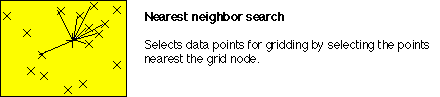## Search algorithms

One critical difference between various local-fit algorithms for interpolating to a regular grid is the way in which "nearest neighbors" are defined and found. Because some search techniques may be superior to others in certain situations (primarily reflecting the arrangement of the data points), Surface III provides a variety of search procedures that may be selected by the user. The simplest method finds the n nearest neighboring data points, in a Euclidean distance sense, regardless of their angular distribution around the grid node being estimated. This method is fast and satisfactory if observations are distributed in a comparatively uniform manner, but provides poor estimates if the data are closely spaced along widely separated traverses.An objection to a simple nearest neighbor search is that all the nearest points may lie in a narrow wedge on one side of the grid node. The resulting estimate of the node is essentially unconstrained, except in one direction. This may be avoided by restricting the search in some way which ensures that the control points are equitably distributed about the grid node being estimated.

The simplest method that introduces a measure of radial is a quadrant search. Some minimum number of control points must be taken from each of the four quadrants around the grid node being calculated. An elaboration on the quadrant search is an octant search, which introduces a further constraint on the radial distribution of the points used in the estimating equation. A specified number of control points must be found in each of the 45 degrees segments surrounding the grid node being estimated. This search method is one of the more elegant procedures and is widely used in commercial contouring programs.These constrained search procedures require finding and testing more neighboring control points than in a simple search, which increases the time required. Surface III also provides an entirely different type of search procedure which finds all points within a radius r of the point being estimated. Estimates made using the other search procedures are based on a fixed number of points collected at variable distances from the grid node; this search algorithm uses a variable number of points found within a fixed distance of the node.

Any constraints on the search for the nearest control points, such as a quadrant or octant requirement, will obviously expand the size of the neighborhood around the grid node being estimated. This occurs because some nearby control points are likely to be passed over in favor of more distant points in order to satisfy the requirement that only a few points may be taken from a single sector. Unfortunately, the autocorrelation of a surface decreases with increasing distance, so these more remote control points are less closely related to the location being estimated. This means the estimate may be poorer than if a simple nearest neighbor search procedure were used.go back